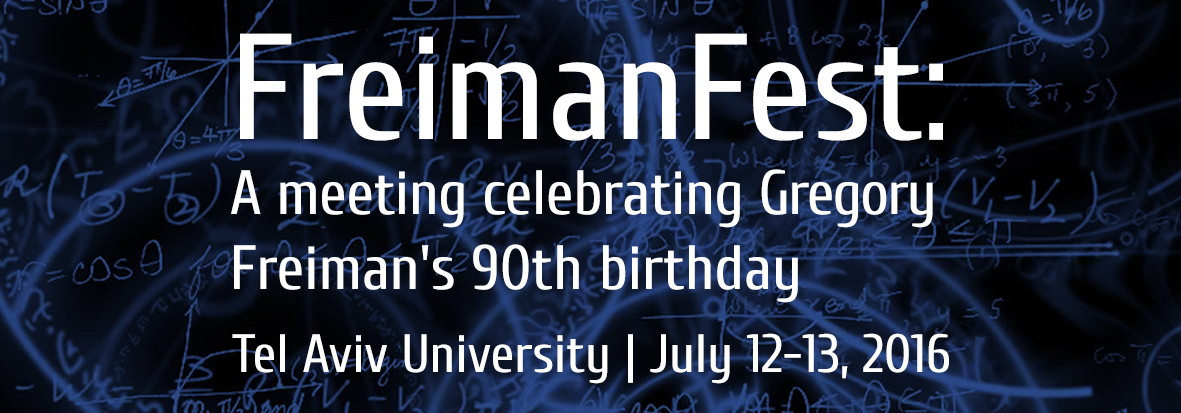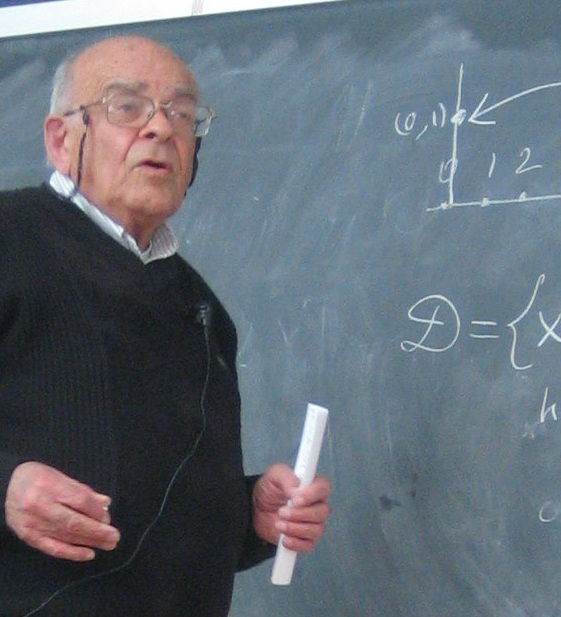﻿

FreimanFest: A meeting celebrating Gregory Freiman's 90th birthday# Schedule

• July 12
• 09:30-10:00: Coffee
• 10-10:45: Oriol Serra
• 11-11:45: Melvyn Nathanson
• 12:00-13:30: Lunch
• 14:00-14:45: Yonutz Stanchescu
• 15:00-15:45: Mercede Maj and Patrizia Longobardi
• 15:45-16:15: Coffee

• July 13
• 09:30-10:00: Coffee
• 10-10:45: Endre Szemerèdi
• 11-11:45: Vsevolod Lev
• 12:00-13:30: Lunch
• 14:00-14:45: Jean-Marc Deshouillers
• 15:00-15:45: Emmanuel Breuillard
• 15:45-16:15: Coffee
• 16:15-16:45: Gregory Freiman and closing remarks

# List of speakers

• Emmanuel Breuillard, Munster
• Jean-Marc Deshouillers, Bordeaux
• Vsevolod Lev, Haifa
• Patrizia Longobardi, Salerno
• Mercede Maj, Salerno
• Melvyn Nathanson, New York
• Oriol Serra, Barcelona
• Yonutz Stanchescu, Tel Aviv
• Endre Szemerèdi, Budapest

• # Titles and Abstracts

Oriol Serra, Universitat Politecnica de Catalunya, Barcelona, Spain
Title: Doubling and Volume: A conjecture of Freiman

Abstract: By the well-known Freiman-Ruzsa theorem, a set A of integers with small doubling, |A+A| \leq c|A|, is a dense subset of a multidimensional arithmetic progression. The search for efficient quantitative estimations of the density of A in the progression in terms of the doubling constant c has been the object of intense research by several authors, including Freiman, Ruzsa, Bilu, Chang, Sanders and Konyagin. Schoen obtained an asymptotic bound which is essentially best possible.

An approach to the problem is to determine the maximum volume of a set with given cardinality and doubling. The dimension of A is the largest dimension of an integer lattice containing a Freiman isomorphic copy of A not contained in a hyperplane. The additive volume of a d-dimensional set A is defined as the smallest cardinality of the convex hull of sets isomorphic to A in the d-dimensional lattice. Freiman conjectured in 2008 a formula for the value of this maximum volume.

The talk will discuss recent advancements in this direction, in particular proving the conjecture for the class of so-called chains. Some extensions and implications will be also discussed.

Joint work with G. A. Freiman.

Melvyn Nathanson, CUNY, New York, USA
Title: Every finite subset of an abelian group is an asymptotic approximate group

Abstract: If $A$ is a nonempty subset of an additive abelian group $G$, then the $h$-fold sumset is

hA = \{x_1 + \cdots + x_h : x_i \in A_i \text{ for } i=1,2,\ldots, h\}.

We do not assume that $A$ contains the identity, nor that $A$ is symmetric, nor that $A$ is finite. The set $A$ is an $(r,\ell)$-approximate group in $G$ if there exists a subset $X$ of $G$ such that $|X| \leq \ell$ and $rA \subseteq XA$. The set $A$ is an asymptotic $(r,\ell)$-approximate group if the sumset $hA$ is an $(r,\ell)$-approximate group for all sufficiently large $h$.

It is proved that every polytope in a real vector space is an asymptotic $(r,\ell)$-approximate group, that every finite set of lattice points is an asymptotic $(r,\ell)$-approximate group, and that every finite subset of every abelian group is an asymptotic $(r,\ell)$-approximate group.

Preprints are posted on arXiv: 1512.03130 and 1512.06478.

Yonutz Stanchescu, Afeka-Tel Aviv Academic College, Israel
Title: Small doubling in Baumslag-Solitar groups and dilates of integers

Abstract: We will present a connection between abelian sumsets estimates and growth in non-abelian groups. More precisely, we investigate small doubling problems in Baumslag-Solitar groups using new direct and inverse results for sums of dilates of integers.

Joint work with G. A. Freiman, M. Herzog, P. Longobardi and M. Maj.

Mercede Maj and Patrizia Longobardi, University of Salerno, Italy
Title: On some Freiman's problems concerning products of subsets in groups

Abstract: Let G denote an arbitrary group. If S is a subset of G, we define its square S^2 by

S^2={ x_1x_2 : x_1,x_2 in S}.

In this talk we are interested in two problems, proposed by Gregory Freiman, concerning products of finite subsets in groups.

First we investigate the class DS(m), with m > 1 an integer, where a group G belongs to DS(m) if the cardinality of S^2 is less that m^2 for every subset S of G of order m.

Then we will present some recent results, obtained jointly with G.A. Freiman, M. Herzog, Y.V. Stanchescu, A. Plagne and D.J.S. Robinson concerning the structure of finite subsets S of an orderable group satisfying the inequality:

|S^2| \leq \alpha|S| + \beta, with \alpha = 3 and small values of |\beta|.

Endre Szemerèdi, Renyi Institute, Budapest, Hungary
Title: Applications of the fundamental theorem of Freiman

Abstract: We are going to discuss some applications of Freiman's theorem in subset-sum problems. We are going to give estimations and structural results.

Vsevolod Lev, University of Haifa, Israel
Title: Quadratic residues and difference sets

Abstract: It has been conjectured by Sarkozy that with finitely many exceptions, the set of quadratic residues modulo a prime $p$ cannot be represented as a sumset $\{a+b\colon a\in A, b\in B\}$ with non-singleton $A,B\subset F_p$. The case $A=B$ of this conjecture has been established by Shkredov. The analogous problem for differences remains open: is it true that for all sufficiently large primes $p$, the set of quadratic residues modulo $p$ is not of the form $\{a'-a''\colon a',a''\in A,\,a'\ne a''\}$ with $A\subset F_p$?

We present the results of our recent paper, joint with Jack Sonn, where a presumably more tractable variant of this problem is considered: is there a set $A\subset F_p$ such that every quadratic residue has a *unique* representation as $a'-a''$ with $a',a''\in A$, and no non-residue is representable in this form? We give a number of necessary conditions for the existence of such $A$, involving for the most part the behavior of primes dividing $p-1$. These conditions enabled us to rule out all primes $p$ bigger than 13 and smaller than $10^{20}$ (the primes $p=5$ and $p=13$ being conjecturally the only exceptions).

Jean-Marc Deshouillers, University of Bordeaux, France
Title: Probabilistic methods for Waring's problem: old and new results

Abstract: TBA

Emmanuel Breuillard, Universitat Munster, Germany
Title: The Elekes-Szabo theorem in higher dimension

Abstract: I will discuss the Elekes-Szabo theorem regarding expanding polynomials and its higher dimensional generalization in which a group structure is derived from a combinatorial constraint. In joint work with Hong Wang we determine the possible groups arising this way.

# Organizing Committee

Noga Alon, Jean-Marc Deshouillers, Marcel Herzog, Vsevolod F. Lev, Melvyn Nathanson, Alain Plagne, Oriol Serra, Yonutz Stanchescu